Courses

# AIIMS Full Mock Test 16

## 200 Questions MCQ Test AIIMS Mock Tests & Previous Year Papers | AIIMS Full Mock Test 16

Description
This mock test of AIIMS Full Mock Test 16 for NEET helps you for every NEET entrance exam. This contains 200 Multiple Choice Questions for NEET AIIMS Full Mock Test 16 (mcq) to study with solutions a complete question bank. The solved questions answers in this AIIMS Full Mock Test 16 quiz give you a good mix of easy questions and tough questions. NEET students definitely take this AIIMS Full Mock Test 16 exercise for a better result in the exam. You can find other AIIMS Full Mock Test 16 extra questions, long questions & short questions for NEET on EduRev as well by searching above.
QUESTION: 1

Solution:
QUESTION: 2

Solution:
QUESTION: 3

### A student measures the distance traversed in free fall a body initially at rest in a given time. He uses this data to estimate g, the acceleration due o gravity. If the maximum percentage errors in the measurement of distance and time are e1 and e2 respectively, the percentage error in the measurement of g is :

Solution:
QUESTION: 4

The velocity versus time graph of a body moving in a straight line is shown in fig. The displacement of the body in 5 s is: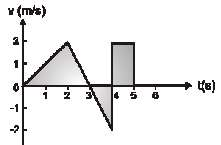Solution:
QUESTION: 5

A projectile is fired at 300 with momentum p. Neglecting air friction, the change n kinetic energy when it returns to the ground will be:

Solution:
QUESTION: 6

A monkey is descending fromthe branch of a tree with acceleration. If the breaking strength of the branch is 75% of the weight of the monkey, the minimum acceleration with which monkey can slide down without breaking the branch is:

Solution:
QUESTION: 7

The coefficient of static friction ( s) between block A of mass 2 kg and the table as shown in Fig. is 0.1. What would be the maximum mass of block B so that the two blocks do not move ? The string and the pulley are assumed to be smooth and mass less (g = 10 m/s2):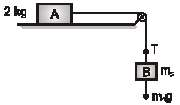Solution:
QUESTION: 8

Two inclined frictionless tracks, one gradual and the steepmeet at A from where two stones are allowed to slide down from rest, one on each track, as shown in Fig. Which of the following statement is correct ?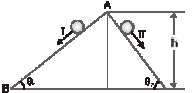Solution:
QUESTION: 9

Two trucks, one loaded (A) and the other unloaded (B) moving and have same kinetic energy. The mass of A is double that of B.Brakes are applied to both and are brought to rest. If the distance covered by A before coming to rest is x1 and that of B is x2 , then:

Solution:
QUESTION: 10

The total work done on a particle is equal to the change in kinetic energy

Solution:
QUESTION: 11

A disc of moment of inertia I1 is rotating with an angular velocity ω1.Another disc of moment of inertia I2 which is not rotating, is gently put on the first disc. The angular speed of the system will be:

Solution:
QUESTION: 12

Two circular discs of same mass and thickness are made from metals having densities ρ1 and ρ2 respectively. The ratio of their moments of inertia a out the central axis perpendicular to the plane of the disc will be:

Solution:
QUESTION: 13

A tangential force F acts along the a ring of radius R and displace the ring through and angle θ . The work done by the force is :

Solution:
QUESTION: 14

If there are no heat losses, the heat released by the condensation of x gram of steam at 1000C into water at 1000C can be used to convert y gram of ice at 00C into water at 1000C. The ratio y : x is nearly

Solution:
QUESTION: 15

Steam at 1000C is passed into 1.1 kg of water contained in a calorimeter of water equivalent 0.02 kg at 150C till the temperature of the calorimeter rises to 800C . The mass of condensed in kg, is:

Solution:
QUESTION: 16

An aluminium sphere of 20cm diameter is heated from 00C to 1000C. Its volume changes by : (Given that coefficient of linear expansion for aluminium, αAl = 23 x 10-6/0C)

Solution:
QUESTION: 17

Two identical disks are positioned on a vertical axis shown in the figure. The bottom disk is rotating at an angular velocity ω0 and has rotational kinetic energy k0. The top disk is initially at rest. It allowed to fall and sticks to the bottom disk The change in the rotational kinetic energy of the system after the collision is: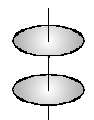Solution:
QUESTION: 18

An ideal monoatomic gas undergoes a process given by P = 3V2. Then

Solution:
QUESTION: 19

An ideal gas heat engine operates in a Carnot cycle be ween 227ºC and 127ºC. It absorbs 6 kcal at the higher temperature. The amount of heat ( n kcal) converted into work is equal to :

Solution:
QUESTION: 20

In a given process on an ideal gas, dW = 0 and dQ < 0 Then for the gas

Solution:
QUESTION: 21

Whenthe net force F acting on the particle varies as its position along x-axis shown in the figure, which of the following cannot be oscillatory motion. (where position of the particle)

Solution:
QUESTION: 22

In SHM, potential energy (U) V/s. time (t), graph is

Solution:
QUESTION: 23

A flywheel rotating about a fixed axis has a kinetic energy of 360 joule when its angular speed is 30 rad/ sec. The moment of inertia of the wheel about the axis of rotation is

Solution:
QUESTION: 24

An object is moving in a circle at constant speed v. The magnitude of rate of change of momentum of the object

Solution:
QUESTION: 25

The following questions consist of two statements one labelled ASSERTION (A) and the another labelled REASON (R). Select the correct answers to these questions from the codes given below :

Assertion : Two blocks of masses m1 and m2 are connected by a light inextensible string (having non zero tension) and this system lies on smooth horizontal surface. The block of mass m1 is pulled to right by horizontal force of magnitude F as shown. Then the value of m1a1 + m2a2 does not depend on tension in the string connecting both the blocks, where a1 and a2 are the magnitudes of acceleration of blocks of masses m1 and m2 respectively.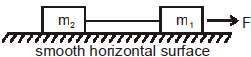Reason : Internal forces with in a system does not depend on net force on the system because sum of all internal forces within a system is zero.

Solution:
QUESTION: 26

The following questions consist of two statements one labelled ASSERTION (A) and the another labelled REASON (R). Select the correct answers to these questions from the codes given below :

Assertion :Anideal gas is enclosedwithin a container fittedwith a piston.When volume of this enclosed gas is increased at constant temperature, the pressure exerted by the gas on the piston decreases.
Reason : In the above situation the rate ofmolecules striking piston decreases. If the rate at which molecules of a gas having same average speed striking a given area of the wall decreases, the pressure exerted by gas on the wall decreases.

Solution:
QUESTION: 27

The following questions consist of two statements one labelled ASSERTION (A) and the another labelled REASON (R). Select the correct answers to these questions from the codes given below :

Assertion : Gas thermometers are more sensitive than liquid thermometers
Reason : Coefficent of thermal expansion of gases is more then liquid

Solution:
QUESTION: 28

The following questions consist of two statements one labelled ASSERTION (A) and the another labelled REASON (R). Select the correct answers to these questions from the codes given below :

Assertion : If direction of net momentum of a system of particles nonzero net momentum  is fixed, the centre of mass of given system moves along a straight line.
Reason : Two particles undergo rectilinear motion along different ght lines. Then the centre of mass of system of given two particles also always moves along a straight line

Solution:
QUESTION: 29

The following questions consist of two statements one labelled ASSERTION (A) and the another labelled REASON (R). Select the correct answers to these questions from the codes given below :

Assertion : Different gases at the same conditions of temperature and pressure have same root mean square speed.
Reason: Average K.E. of gas is inversly proportional to temprature in kelvin

Solution:
QUESTION: 30

The following questions consist of two statements one labelled ASSERTION (A) and the another labelled REASON (R). Select the correct answers to these questions from the codes given below :

Assertion : If resultant of external forces on a system of two spheres is always zero and net momentum of systemof both spheres (undergoing pure translationa lmotion) before undergoing head on collision is zero, then the minimum kinetic energy of system during collision is zero.

Reason : During a headon collision between two bodies; at instant of maximum deformation velocities of both bodies are equal. Also if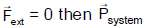= constant.

Solution:
QUESTION: 31

The following questions consist of two statements one labelled ASSERTION (A) and the another labelled REASON (R). Select the correct answers to these questions from the codes given below :

Assertion : The root mean square velocity of molecules of a gas having Maxwellian distribution of velocities is higher than their most probable velocity, at any temperature.
Reason : A very small number of molecules of a gas molecule possess very large velocities

Solution:
QUESTION: 32

The following questions consist of two statements one labelled ASSERTION (A) and the another labelled REASON (R). Select the correct answers to these questions from the codes given below :

Assertion : Fahrenheit is the smallest unit measuring temperature.
Reason : Fahrenheit was the first temperature scale used for measuring temperature.

Solution:
QUESTION: 33

The following questions consist of two statements one labelled ASSERTION (A) and the another labelled REASON (R). Select the correct answers to these questions from the codes given below :

Assertion : If the momentumof a particle is constant, then its angular momentum will also be constant.
Reason :When net external force on an object is zero, then external torque on the object will also be zero.

Solution:
QUESTION: 34

The following questions consist of two statements one labelled ASSERTION (A) and the another labelled REASON (R). Select the correct answers to these questions from the codes given below :

Assertion : Most probable velocity is the velocity possessed by maximum fraction of molecules at the same temperature.
Reason : On collision, more and more molecules acquire higher speed at the same temperature

Solution:
QUESTION: 35

The following questions consist of two statements one labelled ASSERTION (A) and the another labelled REASON (R). Select the correct answers to these questions from the codes given below :

Assertion : A solid sphere and a hollow sphere of same radius and same material are released (at rest) from the top of a fixed inclined plane at the same time. They will reach the bottom simultaneously, if they roll with sliding.
Reason : In the situation of statement-1, the centres of both spheres have the same acceleration and they travel the same distance. Hence time taken is same.

Solution:
QUESTION: 36

The following questions consist of two statements one labelled ASSERTION (A) and the another labelled REASON (R). Select the correct answers to these questions from the codes given below :

Assertion : An ideal mass less spring (K = 10 0 N/cm) is fixed at one end is initially unstretched. An agent slowly stretches the spring from the other end by 1 cm, then work done by agent in stretching the spring will be 0.5 Joule.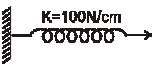Reason :Work done on ideal mass less spring can be stored only in the form of potential energy of the spring.

Solution:
QUESTION: 37

The following questions consist of two statements one labelled ASSERTION (A) and the another labelled REASON (R). Select the correct answers to these questions from the codes given below :

Assertion : A block is moved on rough horizontal fixed surface along a circular path. The work done by force of friction on given rough horizontal surface for the duration in which the block completes a circle is zero.
Reason : For a moving body, if net displacement in a time interval is zero, then the work done by a non zero constant force acting on this body is zero

Solution:
QUESTION: 38

The following questions consist of two statements one labelled ASSERTION (A) and the another labelled REASON (R). Select the correct answers to these questions from the codes given below :

Assertion : The pressure of a gas filled in a cylinder fitted with an immovable piston is increased by raising its temperature.
Reason : On raising the temperature, greater momentum is transferred to the wall of the container per second.

Solution:
QUESTION: 39

The following questions consist of two statements one labelled ASSERTION (A) and the another labelled REASON (R). Select the correct answers to these questions from the codes given below :

Assertion : It is possible for both the pressure and volume of a onoatomic ideal gas of a given amount to change simultaneously without causing the internal energy of the gas to change.
Reason : The internal energy of an ideal gas of a given amount remains constant if temperature does not change. It is possible to have a process in w ch pressure and volume are changed such that temperature remains constant.

Solution:
QUESTION: 40

The following questions consist of two statements one labelled ASSERTION (A) and the another labelled REASON (R). Select the correct answers to these questions from the codes given below :

Assertion : Heat supplied to a system with constant volume is used to increase only the internal energy of the system.
Reason : According to first law of thermodynamics dQ = dU + dW and dW is zero for constant volume.

Solution:
QUESTION: 41

The following questions consist of two statements one labelled ASSERTION (A) and the another labelled REASON (R). Select the correct answers to these questions from the codes given below :

Assertion : Work done by a gas in isothermal expansion is more han the work done by the gas in the adiabatic expansion. (In both cases initial parameters are same and expanded to same final volume)
Reason : Temperature remains constant in isothermal expansion and not in adiabatic expansion.

Solution:
QUESTION: 42

The following questions consist of two statements one labelled ASSERTION (A) and the another labelled REASON (R). Select the correct answers to these questions from the codes given below :

Assertion : Equalmoles of heliumand oxygen gases are given equal quantities of heat at constant volume. There will be a greater rise in the temperature of helium as compared to that of oxygen.
Reason : The molecular weight of oxygen is more than the mole ular weight of helium

Solution:
QUESTION: 43

Which of the following groups exerts significant +M effect as well as –I effect?

Solution:
QUESTION: 44

Inductive effect is a permanent effect and is distance dependent

Solution:
QUESTION: 45

In thermodynamics, a process is called reversible when -

Solution:
QUESTION: 46

Look at the following diagram :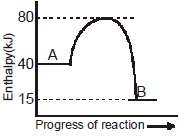The enthalpy change for the reaction A → B will be

Solution:
QUESTION: 47

The following questions consist of two statements one labelled ASSERTION (A) and the another labelled REASON (R). Select the correct answers to these questions from the codes given below :

Assertion : Beryllium does not impart a characteristic color to the flame of Bunsen burner.
Reason : Beryllium requires high amount of energy for excitation of electrons

Solution:
QUESTION: 48

The following questions consist of two statements one labelled ASSERTION (A) and the another
labelled REASON (R). Select the correct answers to these questions from the codes given below :

Assertion :Electron withdrawing groups generally increases the stability of carbocation intermediates.
Reason : Carbocations are electron rich species having complete octet at carbon atom.

Solution:
QUESTION: 49

The following questions consist of two statements one labelled ASSERTION (A) and the another labelled REASON (R). Select the correct answers to these questions from the codes given below :

Assertion : Bond length of NO+ is less than NO.
Reason : Bond order of NO is more than NO+

Solution:
QUESTION: 50

The following questions consist of two statements one labelled ASSERTION (A) and the another labelled REASON (R). Select the correct answers to these questions from the codes given below :

Assertion : Solubilities of alkali metal fluorides and carbonates increase down the group.
Reason : Hydration energies of alkali metal halides decrease down the group with increase in size of cations.

Solution:
QUESTION: 51

The following questions consist of two statements one labelled ASSERTION (A) and the another labelled REASON (R). Select the correct answers to these questions from the codes given below :

Assertion : Benzoate ion is less stable than phenoxide ion.
Reason : In benzoate ion negative charge is poor or weakly delocalised while in phenoxide ion negative charge is better or more delocalised

Solution:
QUESTION: 52

The following questions consist of two statements one labelled ASSERTION (A) and the another labelled REASON (R). Select the correct answers to these questions from the codes given below :

Assertion :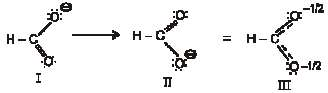I and II are resonating structures and III is resonance hybrid.

Reason : The resonance hybrid is the hypothetical structure that is considered to be a ‘blend’ of the
real contributing structures.

Solution:
QUESTION: 53

The following questions consist of two statements one labelled ASSERTION (A) and the another labelled REASON (R). Select the correct answers to these questions from the codes given below :

Assertion : Heterolytic fission involves the breaking of a covalent bond in such a way that both the electrons of the shared pair are carried away by one of the atoms.
Reason : Heterolytic fission occurs readily in polar covalent bonds.

Solution:
QUESTION: 54

The following questions consist of two statements one labelled ASSERTION (A) and the another labelled REASON (R). Select the correct answers to these questions from the codes given below :

Assertion : The resonating structure of acylium ion,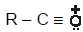is more stable than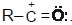Reason : The octet of all atoms is complete in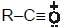Solution:
QUESTION: 55

The following questions consist of two statements one labelled ASSERTION (A) and the another labelled REASON (R). Select the correct answers to these questions from the codes given below :

Assertion : Resonance energy of phenanthrene is more than anthracene.
Reason : Phenanthrene has more aromatic character and more delocalisation than anthrance

Solution:
QUESTION: 56

The following questions consist of two statements one labelled ASSERTION (A) and the another labelled REASON (R). Select the correct answers to these questions from the codes given below :

Assertion : Inductive effects can operates in both sat rated and unsaturated compounds but mesomeric effects can operate only in unsaturated (specially in conjugated compounds).
Reason : I effects involve the σ bonded electrons while the mesomeric effects involve the π bonds and p-orbitals

Solution:
QUESTION: 57

The following questions consist of two statements one labelled ASSERTION (A) and the another labelled REASON (R). Select the correct answers to these questions from the codes given below :

Assertion : When a gas at high pressure expands against vacuum, the magnitude of work done is maximum.
Reason :Work done in expansion depends upon the pressure inside the gas and increase in volume.

Solution:
QUESTION: 58

The following questions consist of two statements one labelled ASSERTION (A) and the another labelled REASON (R). Select the correct answers to these questions from the codes given below :

Assertion : The magnitude of the work involved in an isothermal expansion is greater than that involved in an adiabatic expansion.
Reason : P–V curve (P on y-axis and V on x-axis) decrease more rapidly for reversible adiabatic expansion compared to reversible isothermal expansion starting from same initial state.

Solution:
QUESTION: 59

The following questions consist of two statements one labelled ASSERTION (A) and the another labelled REASON (R). Select the correct answers to these questions from the codes given below :

Assertion : The change in internal energy is zero in isothermal expension of an ideal gas.
Reason : Work done by the gas in reversible adiabatic expansion of an ideal gas is zero.

Solution:
QUESTION: 60

If onlyFAD is hydrogen acceptor in aerobic respiration instead of NAD+, than howmany total ATPmay generate by the complete oxidation of one mole of glucose

Solution:
QUESTION: 61

A physical quantity A is related to four observables a,b,c and d as : A = a2b3/ √c d . The percentage errors of measurements in a,b,c and d are 1%, 32%, 2%and2%respectively.What is the percentage error in the quantity A ?

Solution:
QUESTION: 62

The acceleration a of a particle starting from rest varies with time according to relation : a = αt + β. The velocity of the particle after a time t will be:

Solution:
QUESTION: 63

If two stones projected from the same point with the same speed but at angle π/3 and π/6 respectively have their ranges R2 and R1, then:

Solution:
QUESTION: 64

A ball is let fall from a height h0. There are n collisions with the Earth. If the velocity of rebound after n collisions is vn and the ball rises to a height hn , then coefficient of restitution is given by:

Solution:
QUESTION: 65

A rod of length L and mass M is bent to form a semicircular ring. The moment of inertia of the ring about is diameter is:

Solution:
QUESTION: 66

The figure given below show a process (relating pressure P and volume V) for a given amount for an ideal gas. W is work done by the gas and Q is heat absorbed by the gas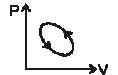Solution:
QUESTION: 67

An ideal gas with pressure P volume V and temperature is expanded isothermally to a volume 2V and a final pressure Pi . If the same gas is expanded adiabatically to a volume 2V, the final pressure is Pa. The ratio of the specific heat of the gas is 1.67. The ratio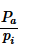is

Solution:
QUESTION: 68

Masses are suspended in vertical plane as shown (the system is at rest and in equilibrium). When m1 is removed the system makes 20 oscillations in 40 sec.When m2 is also removed gently then how long the system will take to complete 30 oscillations :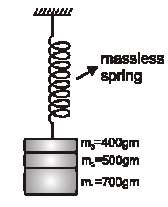Solution:
QUESTION: 69

A particle of mass 10 gm is placed in a potential field given by V= (50 x2 + 100) J/kg. The frequency of oscillation in cycle/sec is :

Solution:
QUESTION: 70

The volume thermal expansion coefficient of an ideal gas at constant pressure is (Here T = absolute temperature of gas)

Solution:
QUESTION: 71

If n1 moles of a monoatomic gas and n2 moles of a diatomic gas are mixed. Then g =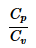mixture is :

Solution:
QUESTION: 72

The maximum velocity of a harmonic oscillator is α and its maximum acceleration is β . Its time period will be.

Solution:
QUESTION: 73

Amplitude of harmonic oscillator is a, when velocity of particle is half ofmaximumvelocity, then position of particle will be

Solution:
QUESTION: 74

The equation of S.H.M. of a particle is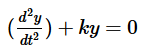, where k is a positive constant. The time period of motion is given by

Solution:
QUESTION: 75

The total energy of the body executing S.H.M. is E. Then the kinetic energy when the displacement is half of the amplitude, is

Solution:
QUESTION: 76

Two semicircular rings of linear mass densities λ and 2λ and of radius ‘R’ each are joined to form a complete ring. The distance of the center of the mass of complete ring from its geometrical centre is :

Solution:
QUESTION: 77

The following questions consist of two statements one labelled ASSERTION (A) and the another labelled REASON (R). Select the correct answers to these questions from the codes given below :

Assertion : The ratio of  Cp/Cv  for an ideal diatomic gas is more than that for an ideal monoatomic gas(where Cp and Cv have usual meaning).
Reason : The atoms of a monoatomic gas have more degrees of freedom as compared to molecules of the diatomic gas.

Solution:
QUESTION: 78

The following questions consist of two statements one labelled ASSERTION (A) and the another labelled REASON (R). Select the correct answers to these questions from the codes given below :

Assertion :Two different gases having same temperature always have molecules with same r.m.s speed.
Reason : The average translational KE per mole for each gas i = 3/2KT (where K = Boltzmann constant, T = temperature in kelvin)-

Solution:
QUESTION: 79

Lone pair on N atom is in conjugated with -electron system in which of the following?

Solution:
QUESTION: 80

Which of the following species is stable at room temperature :

Solution:
QUESTION: 81

In howmany species positive charge is not delocalized ?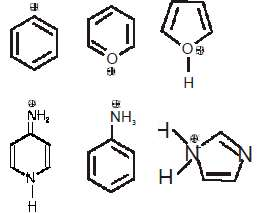Solution:
QUESTION: 82

Which of the following is not aromatic cation ?

Solution:
QUESTION: 83

Maximum –(I)  effect is exerted by the group :

Solution:
QUESTION: 84

The most and least stable anions from the following are respectively :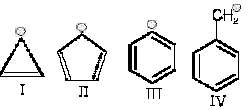Solution:
QUESTION: 85

Which one of the following alkali metals gives hydrated salts ?

Solution:
QUESTION: 86

Which one of the alkaline earth metal carbonates is thermally most stable ?

Solution:
QUESTION: 87

The correct set/s of order is/are
(i) LiCl < BeCl2 < BCl3 < CCl4 (Covalent character )
(ii) Be(OH)2 < Mg(OH)2 < Ca(OH)2 < Sr(OH)2 < Ba(OH)2 (water solubility )
(iii) XeF4 < H2O < NH3 < BF3 (bond angle)
(iv) sp3 < sp2 < sp (% s–character, )

Solution:
QUESTION: 88

Respective order of strength of back-bonding and Lewis acidic strength in boron trihalides is :

Solution:
QUESTION: 89

The correct order of boiling point is :

Solution:
QUESTION: 90

S1 : The HOMO in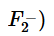is π2pX = π2pY molecular orbitals.
S2 : Bond order of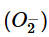is more then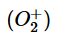S3 :  NO+ is more stable than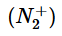S4 :  C2 is more stable than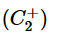State, in order, whether S1, S2, S3, S4 are true or false

Solution:
QUESTION: 91

Which is correct statement about (SiH3)3N is

Solution:
QUESTION: 92

Select the correct statement with respect to alkali metals.

Solution:
QUESTION: 93

The following compounds have been arranged in order of their increasing thermal stabilities. Identify the correct order.
K2CO3 (I ),
MgCO3 (II ),
CaCO3 (III ),
BeCO3( IV)

Solution:
QUESTION: 94

Alkali metals are not characterized by

Solution:
QUESTION: 95

Highly pure dilute solution of sodium in liquid ammonia

Solution:
QUESTION: 96

Maximum – I effect is exerted by the group ?

Solution:
QUESTION: 97

Decreasing + m-power of given group is :
(I) (-O-CH3)  (II) (-F)   (III)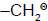(IV) (-Cl)

Solution:
QUESTION: 98

Identify the incorrect statements

Solution:
QUESTION: 99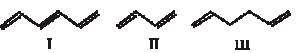Among these compounds, the correct order of resonance energy is :

Solution:
QUESTION: 100

In which of the following molecule, the mesomeric effect is present ?

Solution:
QUESTION: 101

The incorrect statement is :

Solution:
QUESTION: 102

Which is the decreasing order of stability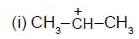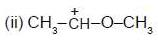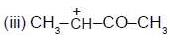Solution:
QUESTION: 103

In which delocalisation of positive charge is possible

Solution:
QUESTION: 104

Two moles of an ideal gas expand spontaneously in vacuum. The work done is

Solution:
QUESTION: 105

The work done in ergs for the reversible expansion of one mole of an ideal gas from a volume of 10 litres to 20 litres at 250C is

Solution:
QUESTION: 106

For the real gases reaction
2CO (g) + O2 (g) → 2CO2 (g) ;
ΔH = – 560 kJ. In 10 litre rigid vessel at 500 K, the initial pressure is 70 bar and after the reaction it becomes 40 bar. The change in internal energy is :

Solution:
QUESTION: 107

For the reaction of one mole of zinc dust with one mole of sulphuric acid in a bomb calorimeter, U and w correspond to

Solution:
QUESTION: 108

In an adiabatic expansion of an ideal gas

Solution:
QUESTION: 109

The temperature of the system decreases in an

Solution:
QUESTION: 110

Acertainmass of gas is expanded from(1L, 10 atm) to (4L, 5 atm) againsta constantexternal pressure of 1 atm. If initial temperature of gas is 300 K and t e heat capacity of process is 50 J/°C. Then the enthalpy change during the process is (1L atm ~ 100 J)

Solution:
QUESTION: 111

The difference between ΔH and ΔE(on amolar basis) for the combustion of n-octane (l) at 250C would be:

Solution:
QUESTION: 112

Equal volumes of molar hydrochloric acid and sulphuric acid are neutralized by dil. NaOH solution and x kcal and y kcal of heat are liberated respectively. Which of the following is true ?

Solution:
QUESTION: 113

Given ioniz H0 (HCN) = 45.2 kJ mol-1 and Δioniz H0 (CH3COOH) = 2.1 kJmol-1.Which one of the following facts is true ?

Solution:
QUESTION: 114

Which of the reaction not defines mola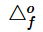?

Solution:
QUESTION: 115

The following questions consist of two statements one labelled ASSERTION (A) and the another labelled REASON (R). Select the correct answers to these questions from the codes given below :

Assertion : Superoxides of alkali metals are paramagnetic.
Reason : Superoxides contain the ion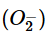which has one unpaired electron in its anti-bonding molecular orbital.

Solution:
QUESTION: 116

The following questions consist of two statements one labelled ASSERTION (A) and the another labelled REASON (R). Select the correct answers to these questions from the codes given below :

Assertion :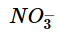and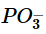have similar formula type but differ structurally i.e. they have different type of hybridisation.
Reason :ion exists as free ion. On the other handexists as cyclic or linear polymeric structure.

Solution:
QUESTION: 117

The following questions consist of two statements one labelled ASSERTION (A) and the another labelled REASON (R). Select the correct answers to these questions from the codes given below :

Assertion : In Allkyl groups + effect order is (CH3)3C– > (CH3)2CH– > CH3–CH2– > CH3– but hyperconjugation effect order is (CH3)3C– < (CH3)3CH– < CH3–CH2– < CH3
Reason : Because both effects are opposite one has electron withdrawing and another has electron donating nature.

Solution:
QUESTION: 118

The following questions consist of two statements one labelled ASSERTION (A) and the another labelled REASON (R). Select the correct answers to these questions from the codes given below :

Assertion : Heat of neutralisation of perchloric acid, HClO4, with NaOH is same as that of HCl with NaOH.
Reason : Both HCl and HClO4 are strong acids.

Solution:
QUESTION: 119

The following questions consist of two statements one labelled ASSERTION (A) and the another labelled REASON (R). Select the correct answers to these questions from the codes given below :

Assertion : In the following reaction : C(s) + O2 (g) → CO2 (g) ; ΔH = ΔU – RT
Reason : ΔH is related to ΔU by the equation, ΔH = ΔU – Δng RT

Solution:
QUESTION: 120

The following questions consist of two statements one labelled ASSERTION (A) and the another labelled REASON (R). Select the correct answers to these questions from the codes given below :

Assertion : Enthalpy of formation of any elemental substance in the standard states is taken as zero.
Reason : Each substance has a definate amount of enthalpy but its exact value can not be calculated

Solution:
QUESTION: 121

The following questions consist of two statements one labelled ASSERTION (A) and the another labelled REASON (R). Select the correct answers to these questions from the codes given below :

Assertion : Many endothermic reactions that are not spontaneous at room temperature become spontaneous at high temperature.
Reason : Enthalpy of reaction may change on changing temperature.

Solution:
QUESTION: 122

Which of the following diagram illustrates the distrib ion of Na+ and K+ ions in a section of nonmyelinated axon

Solution:
QUESTION: 123

Tobacco Mosaic Virus (TMV) was first isolated in crystalline form by –

Solution:
QUESTION: 124

Bacterium whose cells has only a single curve is known as :

Solution:
QUESTION: 125

Eubacteria do not posses :

Solution:
QUESTION: 126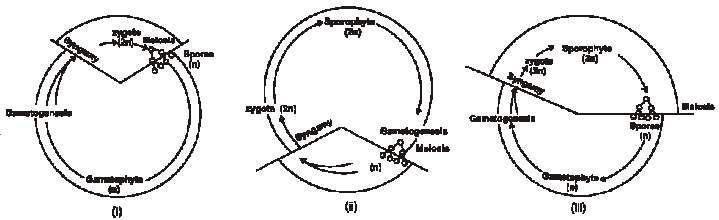Identify (i), (ii), (iii) diagrams & select the correct option with their suitable examples

Solution:
QUESTION: 127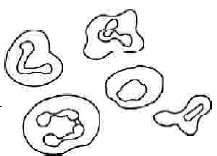The above diagram represents

Solution:
QUESTION: 128

______(i)_______ ismembrane bound space in cytoplasm. Its ______(ii)_______ contains excretory products, water, pigments & is covered by a single membrane called_____(iii)_______ Here (i), (ii), (iii) are respectively

Solution:
QUESTION: 129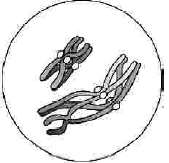This diagram represents

Solution:
QUESTION: 130

In which stage of cell cycle, tetrad is conspicuous

Solution:
QUESTION: 131

Which of the following feature is not related with blue green algae.

Solution:
QUESTION: 132

Archaebacterial cell lack –

Solution:
QUESTION: 133

If a cell has 12 bivalents in zygotene stage howmany kinetochores are found in it.

Solution:
QUESTION: 134

If DNA content of a germinal cell is 2C in G1 stage. What will be the DNA contents in G2 , anaphase-I and meiosis-II respectively

Solution:
QUESTION: 135

...............................is the main area of cellular activities in both the plant and animal cells. Various chemical reactions occur in it to keep the cell in the living state.

Solution:
QUESTION: 136

Select the wrong pair

Solution:
QUESTION: 137

70S type of ribosomes are absent in

Solution:
QUESTION: 138

Solution:
QUESTION: 139

Genetic recombination in bacteria mediated by a virus is known as

Solution:
QUESTION: 140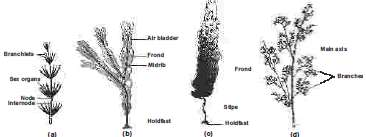In the above diagrams, Identify the correct labelling

Solution:
QUESTION: 141

Linnaeus sexual systemof classification of plants on the basis of number and arrangement of stamens and carpels / morphology of floral parts is

Solution:
QUESTION: 142

Adrenal cortex is composed of 3 layers the sequence of layers from the outside to the inside is

Solution:
QUESTION: 143Identify Ato E

Solution:
QUESTION: 144

Association areas in cerebral cortex are

Solution:
QUESTION: 145

Observe the following figures. Identify A to D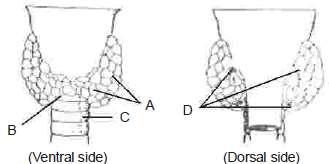Solution:
QUESTION: 146

Photoreceptor cells are present in the ......layer of wall of eye ball

Solution:
QUESTION: 147

Match Column I with Column II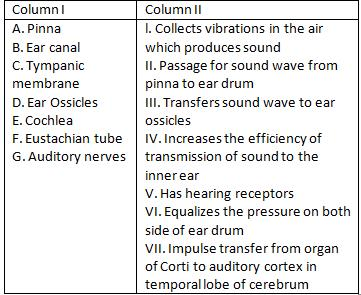Solution:
QUESTION: 148

Sarcomere is the area between -

Solution:
QUESTION: 149

Ca+2  bind _______in the skeletalmuscles and leads to exposure of the binding site for ________on the filament__________

Solution:
QUESTION: 150

Fill in the blank spaces:
(1) A canal called the _____(A)_____passess through the midbrain.
(2) The dorsal protion of the midbrain consists mainly of four round swellings (lobes) called _____(B)_________.
(3) A deep cleft divides the cerebrum longitudinally into two halves, which are termed as the left and right _______(C)_____.
(4) The hemispheres are connected by a tract of nerve fibres called ________(D)________.

Solution:
QUESTION: 151

Column I list the parts of the human brain and column II lists the functions. Match the two columns and identify the correct choice from those given-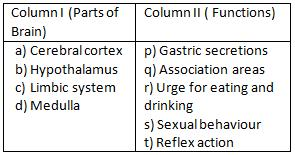Solution:
QUESTION: 152

Fill in the blank spaces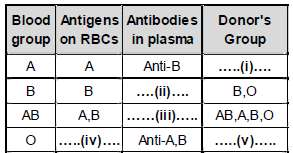Solution:
QUESTION: 153

Observe the above diagramatic representation. Identify A to D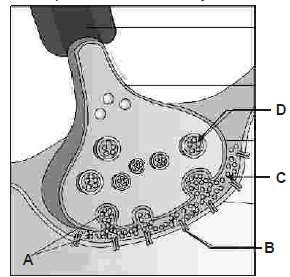Solution:
QUESTION: 154

Go through the following figure -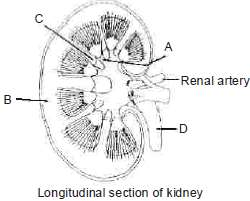IdentifyAto D

Solution:
QUESTION: 155

Match column I with column II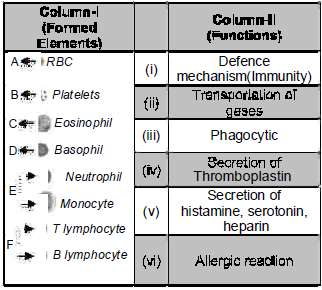Solution:
QUESTION: 156

Match column I with column II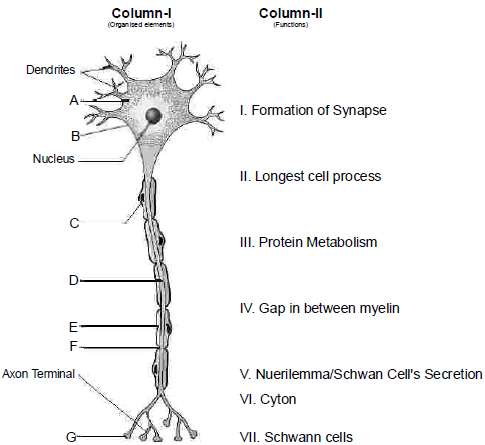Solution:
QUESTION: 157

A man is admitted to a hospital. He is suffering from an abnormally low body temperature, loss of appetite, and exterme thirst.His brain scan would probably show a tumor in

Solution:
QUESTION: 158

Math list I with list II and choose the correct option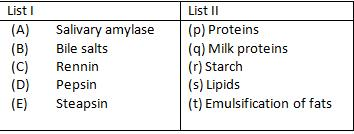Solution:
QUESTION: 159

Match the contents of column-I with those of column-II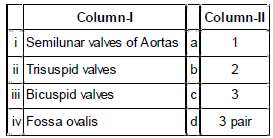Solution:
QUESTION: 160

What is true for Pepsin and Rennin–

Solution:
QUESTION: 161

The following questions consist of two statements one labelled ASSERTION (A) and the another labelled REASON (R). Select the correct answers to these questions from the codes given below :

Asseration : Five kingdom classification is not a phylogenetic classification.
Reason : Monera, protista,plantae and animalia are not placed as per sequence of their origin

Solution:
QUESTION: 162

The following questions consist of two statements one labelled ASSERTION (A) and the another labelled REASON (R). Select the correct answers to these questions from the codes given below :

Assertion. Plasmids are strands of extra chromosomal DNA
Reason. Plastids are found in eukaryotic cells

Solution:
QUESTION: 163

The following questions consist of two statements one labelled ASSERTION (A) and the another labelled REASON (R). Select the correct answers to these questions from the codes given below :

Assertion :Both bryophytes and pteridophytes contain ll-developedmale sex organ called archegonia.
Reason : Biflagellate sperms are formed by their archegonia.

Solution:
QUESTION: 164

The following questions consist of two statements one labelled ASSERTION (A) and the another labelled REASON (R). Select the correct answers to these questions from the codes given below

Assertion :Deuteromycetes are commonly known as imperfect fungi.
Reason : In deuteromycetes only asexual and vegetative phase are known.

Solution:
QUESTION: 165

The following questions consist of two statements one labelled ASSERTION (A) and the another labelled REASON (R). Select the correct answers to these questions from the codes given below :

Assertion : Archaebacteria are most resistant to adverse environmental conditions.
Reason : In archaebacteria, cell mambrane are highly complex.

Solution:
QUESTION: 166

The following questions consist of two statements one labelled ASSERTION (A) and the another labelled REASON (R). Select the correct answers to these questions from the codes given below :

Assertion :In gymnosperms seeds are naked.
Reason : In gymnosperms seeds are not protected with fruit covering

Solution:
QUESTION: 167

The following questions consist of two statements one labelled ASSERTION (A) and the another labelled REASON (R). Select the correct answers to these questions from the codes given below :

Assertion : Angiosperms are an exceptionally large group of plants occuring inwide range of habitats.
Reason: Inangiosperms seedsare protected by fruit coveringsogetextensivelydispersed by different means

Solution:
QUESTION: 168

The following questions consist of two statements one labelled ASSERTION (A) and the another labelled REASON (R). Select the correct answers to these questions from the codes given below :

Assertion : Fungi prefer to grow in cold and humid areas.
Reason : Low temperature favours the metabolic activities.

Solution:
QUESTION: 169

The following questions consist of two statements one labelled ASSERTION (A) and the another labelled REASON (R). Select the correct answers to these questions from the codes given below :

Assertion : Bryophytes are of great ecological importance.
Reason : Bryophytes help in colonisation (succession) on bare rock/soil

Solution:
QUESTION: 170

The following questions consist of two statements one labelled ASSERTION (A) and the another labelled REASON (R). Select the correct answers to these questions from the codes given below :

Assertion : Pteridophytes are not completely successful land plants.
Reason : Pteridophytes are dependent on water for their fertilization.

Solution:
QUESTION: 171

The following questions consist of two statements one labelled ASSERTION (A) and the another labelled REASON (R). Select the correct answers to these questions from the codes given below :

Assertion : Platelets play an important role in blood clotting.
Reason : In the blood oozing froman injury, the platelets disintegrate and release thromboplastin that initiates clotting